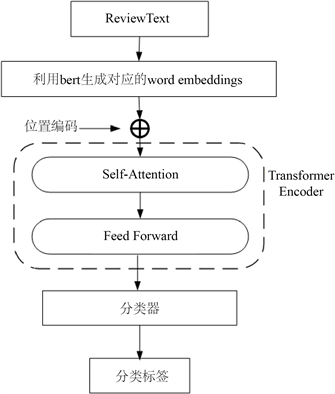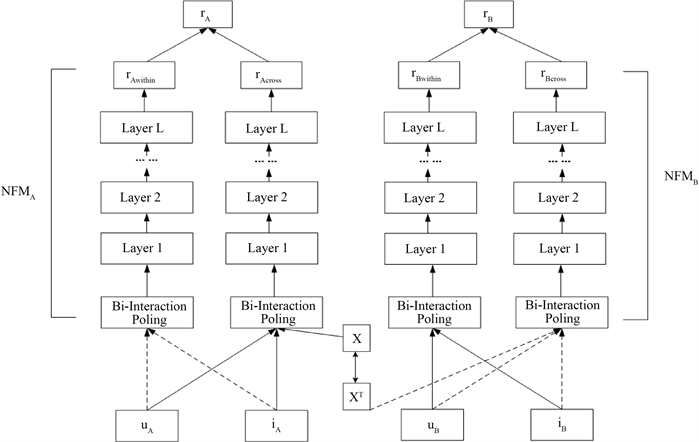﻿ 基于评论情感向量化表示的双向跨域推荐

# 基于评论情感向量化表示的双向跨域推荐Dual Cross Domain Recommendation Based on Sentiment Vectorization of Reviews

Abstract: When most cross-domain recommendation models use review text for cross-domain recommendation, they do not consider the emotional information in the review text. For cross-domain models that take into account the emotional information of comments, most of them are one-way cross-domain recommendations. Therefore, this paper proposes a dual cross-domain recommendation model based on comment sentiment vectorization. The model uses the bert + Transformer method to classify the review emotions of review texts in different fields, and obtains the emotion vectorized representation of the implicit user emotion information, and then calculates the corresponding user preference vector and product feature vector from it, and then cross domain recommendation is carried out according to the generated feature vectors. For cross-domain learning, based on the neural factorization machine (NFM) model, this paper uses the latent orthogonal mapping function to learn the user preferences in the two domains and the user preferences learned across domains, so as to perform two-way recommendation. Through comparative experiments on four datasets, the experimental results show that the model can effectively alleviate the problem of data sparseness.

1. 引言

2. 相关研究

3. 模型设计与分析

3.1. 评论情感信息向量化

bert模型具有多层Transformer  结构，而Transformer是一种基于Attention机制进行特征提取的提取器。从图1中可以看出，Transformer Encoder的结构包括自注意力和前馈神经网络。首先。自注意力对整条评论文本的单词序列进行关注，并帮助模型对单词进行编码，获取到评论文本的上下文语义，再将数据输送到前馈神经网络中进行计算。本文首先通过bert模型将评论文本的单词转换为词向量，再利用Transformer为编码器的方式进行特征提取，并利用分类器进行情感分类。处理流程如图1所示：

$PE\left(pos,2i\right)=\mathrm{sin}\left(pos/{10000}^{2i/{d}_{model}}\right)$ (1)Figure 1. Sentiment classifier

$PE\left(pos,2i+1\right)=\mathrm{cos}\left(pos/{10000}^{2i/{d}_{model}}\right)$ (2)

$u=\frac{{\sum }_{i}vecto{r}_{ui}}{len\left({I}_{u}\right)},i\in {I}_{u}$ (3)

$i=\frac{{\sum }_{i}vecto{r}_{ui}}{len\left({U}_{i}\right)},u\in {U}_{i}$ (4)

3.2. 基于NFM的跨域学习

${\stackrel{^}{r}}_{A}=\left(1-\alpha \right){\text{NFM}}_{A}\left({u}_{A},{i}_{A}\right)+\alpha {\text{NFM}}_{B}\left(X\ast {u}_{A},{i}_{A}\right)$ (5)

${\stackrel{^}{r}}_{B}=\left(1-\alpha \right){\text{NFM}}_{B}\left({u}_{B},{i}_{B}\right)+\alpha {\text{NFM}}_{A}\left({X}^{\text{T}}\ast {u}_{B},{i}_{B}\right)$ (6)Figure 2. Potential orthogonal mapping on NFM model

$x=u\ast v$ (7)

NFM模型的定义公式如下：

$\stackrel{^}{r}\left(x\right)={w}_{0}+\underset{i=1}{\overset{n}{\sum }}{w}_{i}{x}_{i}+f\left(x\right)$ (8)

${f}_{BI}\left(x\right)=\frac{1}{2}\left[{\left(\underset{i=1}{\overset{n}{\sum }}{x}_{i}{v}_{i}\right)}^{2}-\underset{i=1}{\overset{n}{\sum }}{\left({x}_{i}{v}_{i}\right)}^{2}\right]$ (9)

$\stackrel{^}{r}\left(x\right)={w}_{0}+\underset{i=1}{\overset{n}{\sum }}{w}_{i}{x}_{i}+{h}^{\text{T}}{\sigma }_{L}\left({W}_{L}\left(\cdots {\sigma }_{1}\left({W}_{1}{f}_{BI}\left({V}_{x}\right)+{b}_{1}\right)\cdots \right)+{b}_{L}\right)$ (10)

3.3. 损失函数和目标函数

$l\left(y,\stackrel{^}{y}\right)=y\mathrm{log}\stackrel{^}{y}+\left(1-y\right)\mathrm{log}\left(1-\stackrel{^}{y}\right)$ (11)

$\mathrm{min}|{V}_{A}-{\stackrel{^}{r}}_{A}|+|{V}_{B}-{\stackrel{^}{r}}_{B}|$ (12)

4. 实验方案与结果分析

4.1. 数据集Table 1. Dataset statistics table

4.2. 数据预处理和参数设计

4.3. α的取值Figure 3. MSE values in different parameter α

4.4. 对比模型

$\text{MSE}=\frac{1}{N}{\sum }_{n=1}^{N}{\left({\stackrel{^}{r}}_{ui}-{r}_{ui}\right)}^{2}$ (11)

1) FM：一种基于矩阵分解的模型，可以解决由数据稀疏引起的特征组合问题。

2) NFM：在FM模型上，引入Bi-Interaction pooling进行评分预测。

3) DDTCDR：该模型在NCF模型的基础上利用潜在正交映射进行跨域推荐。根据文献描述，在α取0到0.2之间的正值，模型的效果最好。在该模型中，本文把α值设为0.03。

4.5. 实验结果和分析Table 2. Comparison of the effects of each model in different datasets

FM模型和NFM模型进行对比，可以发现NFM模型在特征提取的能力比FM强。将FM模型和RSCDR模型进行比较，可以观察到RSCDR的性能提升不少。

RSCDR模型基于NFM模型，通过进行对比，RSCDR在三个数据组中取得最优结果，在“Movie-Book”实验组中，RSCDR模型和NFM模型在Movie数据集结果相差很小，但在同组的Book数据集中，RSCDR模型比NFM提高了2.1%。同时，在“Movie-Music”数据组中，RSCDR分别提高0.64%和1.48%，在“Book-Music”和“Android-Toy”数据组中，RSCDR比NFM模型分别提高了2.33%、2.61%、0.74%和2.16%，由此可见，利用潜在正交矩阵进行跨域推荐RSCDR模型能够有效解决推荐系统中数据稀疏的问题。

DDTCDR模型与本文模型最为相似，都是利用了潜在正交矩阵进行跨域映射，进行双向推荐。但从本文实验结果上看，只有在“Android-Toy”数据组的Toy数据集中，RSCDR模型的性能比不上DDTCDR模型，但在其余三组数据组中，DDTCDR模型取得的效果并不明显，RSCDR的MSE值均能比DDTCDR要低。因此，在本实验中，从总体上说明基于NFM的RSCDR模型能有效挖掘出交互特征的深层含义，能够提高跨域推荐的性能。

5. 结束语

 Cantador, I., Fernández-Tobías, I., Berkovsky, S. and Cremonesi, P. (2015) Cross-Domain Recommender Systems. In: Recommender Systems Handbook, Springer, Berlin, 919-959.
https://doi.org/10.1007/978-1-4899-7637-6_27

 Xu, Y., Peng, Z., Hu, Y., et al. (2018) SARMF: A Senti-ment-Aware Review Feature Mapping Approach for Cross- Domain Recommendation. International Conference on Web Information Systems Engineering, Dubai, 12-15 November 2018, 3-18.
https://doi.org/10.1007/978-3-030-02925-8_1

 Fu, W.J., Peng, Z.H., Wang, S.Z., et al. (2019) Deeply Fusing Reviews and Contents for Cold Start Users in Cross- Domain Recommendation Systems. Proceedings of the AAAI Con-ference on Artificial Intelligence, 33, 94-101.
https://doi.org/10.1609/aaai.v33i01.330194

 Wang, X.H., Peng, Z.H., Wang, S.Z., et al. (2020) CDLMF: Cross-Domain Recommendation for Cold-Start Users via Latent Feature Mapping. Knowledge and Information Systems, 62, 1723-1750.

 Devlin, J., Chang, M.W., Lee, K., et al. (2018) BERT: Pre-Training of Deep Bidirectional Trans-formers for Language Understanding.

 Vaswani, A., Shazeer, N., Parmar, N., et al. (2017) Attention Is All You Need. Proceedings of Conference of Neural Information Processing Systems, Long Beach, 2017, 6000-6010.

 He, X.G. and Chua, T.S. (2017) Neural Factorization Machines for Sparse Predictive Analytics.
https://doi.org/10.1145/3077136.3080777

 Ruck, D.W. and Rogers, S.K. (1990) The Multilayer Perceptron as an Approximation to a Bayes Optimal Discriminant Function. IEEE Transactions on Neural Networks, 1, 296.
https://doi.org/10.1109/72.80266

 Vinceny, P., Larochelle, H., Lajoie, I., et al. (2010) Stacked Denoising Au-toencoders: Learning Useful Representations in a Deep Network with a Local Denoising Criterion. Journal of Machine Learning Research, 11, 3371-3408.

 蔡泳. 基于评论信息的跨领域商品推荐研究[D]: [硕士学位论文]. 广州: 华南理工大学, 2019.

 Zheng, L., Noroozi, V. and Yu, P.S. (2017) Joint Deep Modeling of Users and Items Using Reviews for Recommendation. Proceedings of the Tenth ACM International Conference on Web Search and Data Min-ing, Cambridge, 6-10 February 2017, 425-434.
https://doi.org/10.1145/3018661.3018665

 Kim, Y. (2014) Convolutional Neural Networks for Sentence Classification. Proceedings of the 2014 Conference on Empirical Methods in Natural Language Processing, Doha, 25-29 October 2014, 1746-1751.
https://doi.org/10.3115/v1/D14-1181

 Caruana, R. (1997) Multitask Learning. Machine Learning, 28, 41-75.
https://doi.org/10.1023/A:1007379606734

 Rendle, S. (2010) Factorization Machines. IEEE International Conference on Data Mining, Sydney, 13-17 December 2010, 995-1000.
https://doi.org/10.1109/ICDM.2010.127

 Zhu, F., Chen, C., Wang, Y., et al. (2019) DTCDR: A Framework for Dual-Target Cross-Domain Recommendation. The 28th ACM International Conference, Huntsville, 27-30 October 2019, 1533-1542.
https://doi.org/10.1145/3357384.3357992

 He, X.N., Liao, L.Z., Zhang, H.W., Nie, L.Q., Hu, X. and Chua, T.-S. (2017) Neural Collaborative Filtering. In: Proceedings of the 26th International Conference on World Wide Web (WWW = 17), International World Wide Web Conferences Steering Committee, Geneva, 173-182.

 Li, P. and Tu-zhilin, A. (2019) DDTCDR: Deep Dual Transfer Cross Domain Recommendation.
https://doi.org/10.1145/3336191.3371793

 Hyun, D., Park, C., Yang, M.C., Song, I., Lee, J.T. and Yu, H. (2018) Review Sentiment-Guided Scalable Deep Recommender System.
https://doi.org/10.1145/3209978.3210111

 Mikolov, T., Chen, K., Corrado, G., et al. (2013) Efficient Estima-tion of Word Representations in Vector Space.

 McAuley, J., Pandey, R. and Leskovec, J. (2015) Inferring Net-works of Substitutable and Complementary Products. Proceedings of the 21st ACM SIGKDD International Conference on Knowledge Discovery and Data Mining, Sydney, 10-13 August 2015, 785-794.
https://doi.org/10.1145/2783258.2783381

 Sun, Z., Yu, D., Fang, H., Qu, X., Zhang, J. and Geng, C. (2020) Are We Evaluating Rigorously? Benchmarking Recommendation for Reproducible Evaluation and Fair Comparison. RecSys’20: Fourteenth ACM Conference on Recommender Systems, New York, 23-32.
https://doi.org/10.1145/3383313.3412489

Top